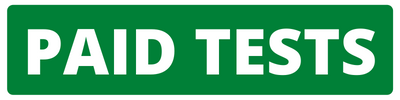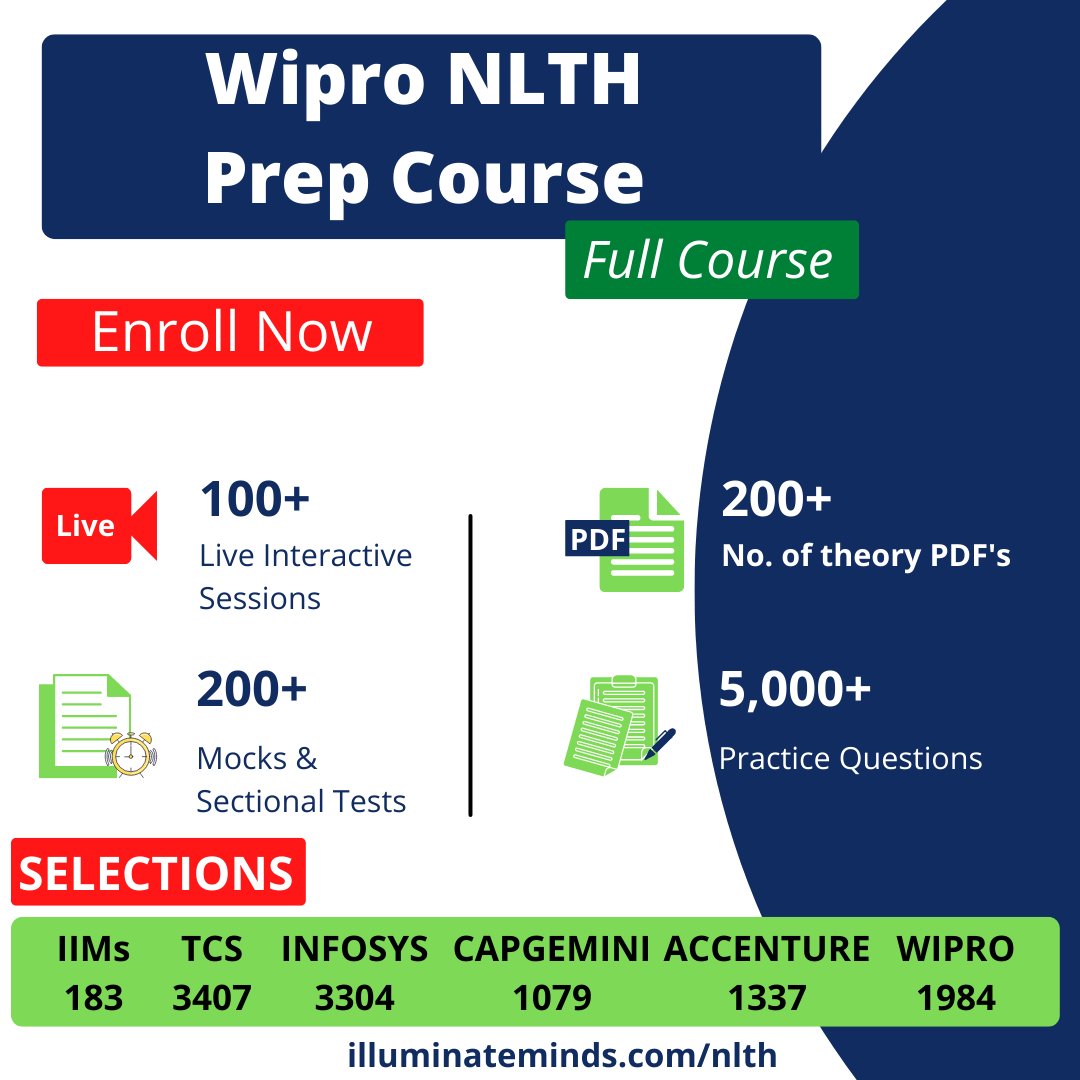## Cognizant Quants QuestionsJobs By Batch
Jobs By Location
Jobs By Degree
Jobs By Branch
IT Jobs
Internships
Govt. Jobs• ##### Original Questions of IT Companies| All Topics### Quiz Begins Here

Q #1
:

#### Cognizant Quants Question

A jar contains 5 white, 8 red, 2 blue and 3 black balls. Find the probability that a ball drawn at random is red or blue.
+

Total number of balls in the bag = 5 + 8 + 2 + 3 = 18

Number of red or blue balls in the bag = 8 + 2 = 10

Probability = 1018 = 59

Q #2
:

#### Cognizant Quants Question

Convert 4.3333��.. into p/q form
+

Let x = 4.333…… (1)

Multiply both sides by 10

10x = 43.333…… (2)

Subtract (1) from (2)

9x = 39

x = 399

Q #3
:

#### Cognizant Quants Question

A vendor purchases binder clips at 12 for Rs. 60. How many clips should he sell for Rs. 60 to earn a profit of 20%?
+

Cost of 12 clips = Rs. 60

Profit = 20%

Selling Price of clips = CP x 120100 = 60 x 120100 = Rs. 72

Selling Price of 12 clips = Rs. 72

Rs. 72 is the selling price of 12 clips.

Rs. 60 will be the selling price of 1272 x 60 = 10 clips

Q #4
:

#### Cognizant Quants Question

The largest number that always divides the difference of a three � digit number and the number formed by reversing its digits is:
+

Let the digits at hundreds, tens and unit place be x, y and z respectively.

The number will be 100x+10y+z

On reversing the positions, number will be 100z+10y+x

On subtracting, we get ((100x+10y+z) – (100z+10y+x)) = 99x-99z = 99(x-z)

The largest number dividing the difference is 99.

Q #5
:

#### Cognizant Quants Question

There are four prime numbers written in ascending order of magnitude. The product of the first three is 385 and that of the last three is 1001. Find the first number.
+

Factors of 385 = 5 * 7 * 11

Factors of 1001 = 7 * 11 *13

First prime number = 5